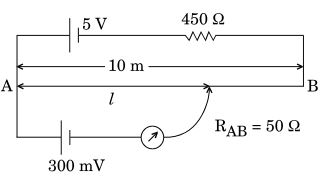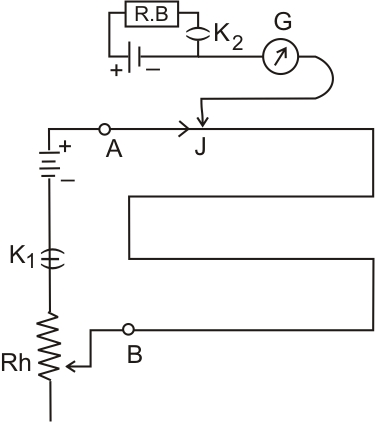# (a) Describe briefly, with the help of circuit diagram, the method of measuring the internal resistance of a cell.(b) Give the reason why a potentiometer is preferred over a voltmeter for measurement of emf of a cell.(c) In the potentiometer circuit given below, calculate the balancing length l. Give reasons, whether the circuit will work, if driver cell of emf 5V is replaced with a cell 2V, keeping all other factors constant.(a) The internal resistance of the primary cell; close the key K1. A constant current flows through the potentiometer wire.

Keeping K2 key open, move the jockey along AB till it balances the emf of the cell.

Now, let us consider the l1 be the balancing length of the wire. If K is the potential gradient, then emf of the cell will be:Introduce a resistance R and close key K2 . With the help of resistance box R.B. select a resistance such that l2 is the balancing length, thenOn dividing both equations, we haveConsider, r be the internal resistance of cell and I be the current flows through the cell.

From Ohm's law, we getTherefore,Hence internal resistance,(b) The voltmeter draws a small current from the cell for its operation. So, in closed circuit measures the terminal potential difference which is less than the emf of a cell. While potentiometer draws no current from the voltage source being measured

That's the reason, why A potentiometer is preferred over a voltmeter for measuring the emf of a cell.

(c) Calculating the balancing length l.

Such that,Now, we know the potential gradient:and balancing length, L=If the driver cell of emf 5V is replaced with a cell of 2V keeping all other factors constant then potential drop along AB is 0.2Volt.

Since the balance point can't be obtained on the potentiometer if the fall of potential along the potentiometer wire is due to the auxiliary battery is less than the emf of the cell to be measured.

Internal resistance of primary cell; close the key k1.A constant current flows through the potentiometer wire.                                                                                                                Keeping key k2 open.then the jockey is moves on wire AB till the emf of cell balanced.             Now,lets consider L1 is the balanced length and K is potential gradiant , then emf of the cell will be E= KL1 ......(1)                                                                                                                Intoduce the rasistance R and close the key k2. with the help of resistance box select a resistance such that L2 is the balancing length.                                                         V= KL2....(2)                                                                                                                      by 1 and 2 eq                                                                                                                     E/V = KL1/KL2                                                                                                                  V = E - Ir

## Most Viewed Questions

### Preparation Products

##### Knockout CUET (Physics, Chemistry and Mathematics)

Complete Study Material based on Class 12th Syllabus, 10000+ Question Bank, Unlimited Chapter-Wise and Subject-Wise Mock Tests, Study Improvement Plan.

₹ 7999/- ₹ 4999/-
##### Knockout CUET (Physics, Chemistry and Biology)

Complete Study Material based on Class 12th Syllabus, 10000+ Question Bank, Unlimited Chapter-Wise and Subject-Wise Mock Tests, Study Improvement Plan.

₹ 7999/- ₹ 4999/-
##### Knockout JEE Main (Six Month Subscription)

- AI Coach Study Modules, - Unlimited Mock Tests, - Study Improvement Plan.

₹ 9999/- ₹ 8499/-
##### Knockout JEE Main (Nine Month Subscription)

- AI Coach Study Modules, - Unlimited Mock Tests, - Study Improvement Plan.

₹ 13999/- ₹ 12499/-